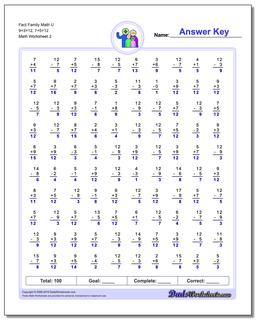# Math Worksheets: Fact Family Math: Fact Family Math: Fact Family Math U 9+3=12, 7+5=12 (Second Worksheet)## Fact Family Math U 9+3=12, 7+5=12 (Second Worksheet)

PropertyValue
DescriptionFact Family Math U 9+3=12, 7+5=12: These math worksheets have 100 addition and subtraction fact family problems and make for a challenging two minute test. (Second Worksheet)
Resource TypeWorksheet